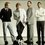# Proof of fermats theorem in some simple way

Fermats theoremNote by Hardik Chandak
6 years, 9 months ago

This discussion board is a place to discuss our Daily Challenges and the math and science related to those challenges. Explanations are more than just a solution — they should explain the steps and thinking strategies that you used to obtain the solution. Comments should further the discussion of math and science.

When posting on Brilliant:

• Use the emojis to react to an explanation, whether you're congratulating a job well done , or just really confused .
• Ask specific questions about the challenge or the steps in somebody's explanation. Well-posed questions can add a lot to the discussion, but posting "I don't understand!" doesn't help anyone.
• Try to contribute something new to the discussion, whether it is an extension, generalization or other idea related to the challenge.

MarkdownAppears as
*italics* or _italics_ italics
**bold** or __bold__ bold
- bulleted- list
• bulleted
• list
1. numbered2. list
1. numbered
2. list
Note: you must add a full line of space before and after lists for them to show up correctly
paragraph 1paragraph 2

paragraph 1

paragraph 2

[example link](https://brilliant.org)example link
> This is a quote
This is a quote
    # I indented these lines
# 4 spaces, and now they show
# up as a code block.

print "hello world"
# I indented these lines
# 4 spaces, and now they show
# up as a code block.

print "hello world"
MathAppears as
Remember to wrap math in $$ ... $$ or $ ... $ to ensure proper formatting.
2 \times 3 $2 \times 3$
2^{34} $2^{34}$
a_{i-1} $a_{i-1}$
\frac{2}{3} $\frac{2}{3}$
\sqrt{2} $\sqrt{2}$
\sum_{i=1}^3 $\sum_{i=1}^3$
\sin \theta $\sin \theta$
\boxed{123} $\boxed{123}$

Sort by:

Are you looking for a simple proof of Fermat's Little Theorem or Fermat's Last Theorem or one of the many other theorems named after Fermat?

- 6 years, 9 months ago

FERMATS LAST THEOREM

- 4 years, 3 months ago

I'm pretty sure Fermat's Last Theorem

- 6 years, 9 months ago

Fermat's Little Theorem can be proved using induction.

- 6 years, 9 months ago

Can u prove it by induction plz show?

- 6 years, 9 months ago

It's not by induction, but an easy proof of Fermat's little theorem would be that $x^p \equiv (1+1+1+...+1)^p \equiv (1^p+1^p+...+1^p) \equiv x \pmod p$ with $x$ times number $1$. This is possible because in Pascal's triangle on prime rows the numbers are multiples of p except for the first and last terms which are $1$. This can be easily proven by using binomial formula.

- 6 years, 9 months ago

Okay get your calculators and try this:

$\sqrt{1782^{12}+1841^{12}}$

$=1922$ right?

So this implies that ${1782^{12}+1841^{12}=1922^{12}}$

Does this disprove Fermat's Last Theorem?

Of course not!

The calculator is wrong.

- 6 years, 9 months ago

BTW: A quick check to see that $1782^{12}+1841^{12} \neq 1922^{12}$ is to note that the left side is odd whereas the right side is even.

- 6 years, 9 months ago

Took some time to realize... The actual answer is $1921.99999995586722540291132837029507293441170657370868230...$ Unfortunately, most calculators round the answer.

- 6 years, 9 months ago

If I remember correctly, that "equation" was from a Homer Simpson episode.

- 6 years, 9 months ago

I can give you a proof .

- 5 years, 7 months ago